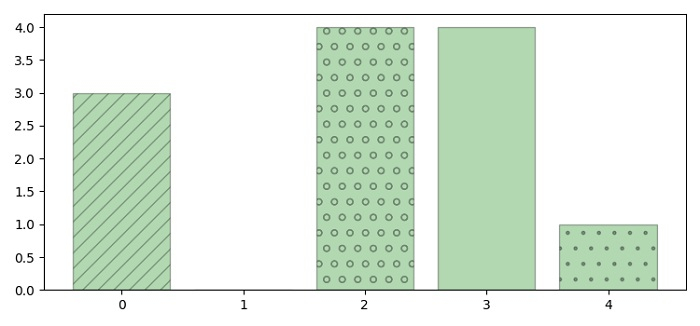# How can I add textures to my bars and wedges in Matplotlib?

To add textures to bars and wedges we can take the following steps −

• Set the figure size and adjust the padding between and around the subplots.

• Create a new figure or activate an existing figure using figure() method.

• Add an axes to the figure as part of a subplot arrangement.

• Make a list of hatches. Bars could be filled with some hatches.

• Create number bars as equivalent to number of hatches.

• Use bar() method to plot the bars with different hatch.

• To display the figure, use show() method.

## Example

import numpy as np
from matplotlib import pyplot as plt

plt.rcParams["figure.figsize"] = [7.50, 3.50]
plt.rcParams["figure.autolayout"] = True

fig = plt.figure()
plt.show()